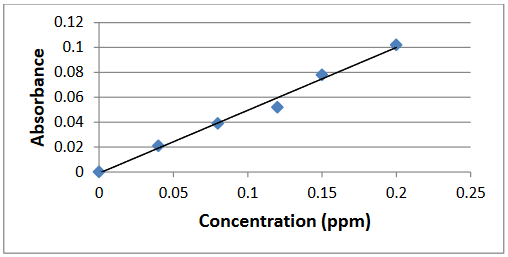# Exploring fundamental relationships in spectrophotometry

This section of the module is designed to provide a review of basic concepts of spectrophotometry. If students have never been introduced to spectrophotometry, a more in depth coverage may be necessary to bring them up to speed, particularly if the module is being used as a lab experiment.

Q1. What wavelength range constitutes the visible region in the electromagnetic spectrum?

The visible region corresponds to wavelengths between 400 and 700 nm.

Q2. Draw a representative plot of A versus c.

Students should draw a typical plot reporting the absorbance on the y-axis and the concentration on the x-axis. A generic plot is presented below.Q3. How could you use this plot to determine the molar extinction coefficient of the analyte being investigated?

According to Beer’s law, A = εbc, where A is the absorbance, ε is the molar extinction coefficient, b is the path length of the cuvette and c is the concentration. Thus, the molar extinction coefficient can be obtained by calculating the slope of the absorbance vs. concentration plot. Since in most instances the path length b of the cuvette is equal to 1 cm, the slope is the same value as ε.

Q4. What color would a particle that absorbs in the blue-green region of the electromagnetic spectrum appear?

The particle would appear red. As showed in Figure 2, gold nanoparticles can appear anywhere from red to purple/blue depending on their size.

Q5. What trend can be identified in the molar extinction coefficient value as particle size increases? How may this trend affect the choice of particle size in a colorimetric sensor?

To answer these questions students should be given time to analyze data provided in Table 1. The table shows that the molar extinction coefficient increases by four orders of magnitude as particle size increases from 5 to 100 nm. Answering the second part of the question may be more challenging. First, the instructor may want to clarify what a colorimetric sensor is. A pregnancy test is a good example. To further guide students, the instructor may want to discuss the significance of the molar extinction coefficient in terms of color intensity. The larger the extinction coefficient, the larger the absorbance for the same concentration of sample. Thus, when developing a colorimetric sensor, particles with larger molar extinction coefficient will produce larger changes in absorbance for the same concentration change, resulting in a more sensitive sensor.